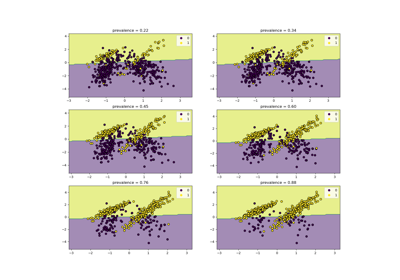# sklearn.metrics.class_likelihood_ratios¶

sklearn.metrics.class_likelihood_ratios(y_true, y_pred, *, labels=None, sample_weight=None, raise_warning=True)[source]

Compute binary classification positive and negative likelihood ratios.

The positive likelihood ratio is LR+ = sensitivity / (1 - specificity) where the sensitivity or recall is the ratio tp / (tp + fn) and the specificity is tn / (tn + fp). The negative likelihood ratio is LR- = (1 - sensitivity) / specificity. Here tp is the number of true positives, fp the number of false positives, tn is the number of true negatives and fn the number of false negatives. Both class likelihood ratios can be used to obtain post-test probabilities given a pre-test probability.

LR+ ranges from 1 to infinity. A LR+ of 1 indicates that the probability of predicting the positive class is the same for samples belonging to either class; therefore, the test is useless. The greater LR+ is, the more a positive prediction is likely to be a true positive when compared with the pre-test probability. A value of LR+ lower than 1 is invalid as it would indicate that the odds of a sample being a true positive decrease with respect to the pre-test odds.

LR- ranges from 0 to 1. The closer it is to 0, the lower the probability of a given sample to be a false negative. A LR- of 1 means the test is useless because the odds of having the condition did not change after the test. A value of LR- greater than 1 invalidates the classifier as it indicates an increase in the odds of a sample belonging to the positive class after being classified as negative. This is the case when the classifier systematically predicts the opposite of the true label.

A typical application in medicine is to identify the positive/negative class to the presence/absence of a disease, respectively; the classifier being a diagnostic test; the pre-test probability of an individual having the disease can be the prevalence of such disease (proportion of a particular population found to be affected by a medical condition); and the post-test probabilities would be the probability that the condition is truly present given a positive test result.

Read more in the User Guide.

Parameters:
y_true1d array-like, or label indicator array / sparse matrix

Ground truth (correct) target values.

y_pred1d array-like, or label indicator array / sparse matrix

Estimated targets as returned by a classifier.

labelsarray-like, default=None

List of labels to index the matrix. This may be used to select the positive and negative classes with the ordering labels=[negative_class, positive_class]. If None is given, those that appear at least once in y_true or y_pred are used in sorted order.

sample_weightarray-like of shape (n_samples,), default=None

Sample weights.

raise_warningbool, default=True

Whether or not a case-specific warning message is raised when there is a zero division. Even if the error is not raised, the function will return nan in such cases.

Returns:
(positive_likelihood_ratio, negative_likelihood_ratio)tuple

A tuple of two float, the first containing the Positive likelihood ratio and the second the Negative likelihood ratio.

Warns:
When false positive == 0, the positive likelihood ratio is undefined.
When true negative == 0, the negative likelihood ratio is undefined.
When true positive + false negative == 0 both ratios are undefined.
In such cases, UserWarning will be raised if raise_warning=True.

References

Examples

>>> import numpy as np
>>> from sklearn.metrics import class_likelihood_ratios
>>> class_likelihood_ratios([0, 1, 0, 1, 0], [1, 1, 0, 0, 0])
(1.5, 0.75)
>>> y_true = np.array(["non-cat", "cat", "non-cat", "cat", "non-cat"])
>>> y_pred = np.array(["cat", "cat", "non-cat", "non-cat", "non-cat"])
>>> class_likelihood_ratios(y_true, y_pred)
(1.33..., 0.66...)
>>> y_true = np.array(["non-zebra", "zebra", "non-zebra", "zebra", "non-zebra"])
>>> y_pred = np.array(["zebra", "zebra", "non-zebra", "non-zebra", "non-zebra"])
>>> class_likelihood_ratios(y_true, y_pred)
(1.5, 0.75)


To avoid ambiguities, use the notation labels=[negative_class, positive_class]

>>> y_true = np.array(["non-cat", "cat", "non-cat", "cat", "non-cat"])
>>> y_pred = np.array(["cat", "cat", "non-cat", "non-cat", "non-cat"])
>>> class_likelihood_ratios(y_true, y_pred, labels=["non-cat", "cat"])
(1.5, 0.75)


## Examples using sklearn.metrics.class_likelihood_ratios¶Class Likelihood Ratios to measure classification performance

Class Likelihood Ratios to measure classification performance